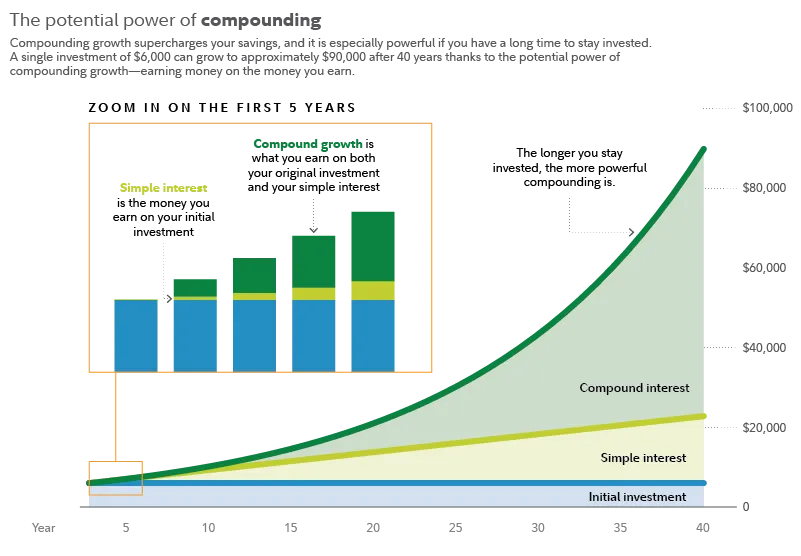top of page

# COMPOUND INTEREST - Why it is 8th Wonder of the World

"My wealth has come from a combination of living in America, some lucky genes, and compound interest." - Warren Buffett

Compound interest — it’s either the easiest way to double or even triple your savings, or a sure-fire ticket to bankruptcy.

Compound interest is different from simple interest. Simple interest is a fixed rate over time, based on the initial amount you’ve invested.Compound Interest - Eight wonder of the world

## Simple Interest

To understand simple interest, let’s assume you deposit \$100 into an account with a 5 percent interest rate. Multiply your principal by the interest rate, and then the amount of time you expect to keep that money in the account.

One hundred dollars times 5 percent, or 0.05, is \$5. Keep that account going for 50 years, and you’ll earn \$250 in interest, for a grand total of \$350.

```P = 100, r =5%, t = 50 years
Simple Interest = (P x r x t)
100 x 5% = 5
5 x 50 = 250```

## Compound Interest

Compound interest is different. It’s interest on top of interest. If you use it correctly, you can turn small initial investments into small fortunes by significantly enhance the growth of your investment portfolio.

Thats why its a common saying power of putting your money to work. By leveraging the concept of compounding, you can harness the potential of your investments and generate substantial wealth over time. The beauty of compounding lies in its ability to create a snowball effect, where your initial investment gains momentum and multiplies exponentially.

Imagine a scenario where your investment not only earns returns on the principal amount but also generates additional returns on those returns. This compounding effect can unlock extraordinary growth opportunities that may seem unattainable otherwise. By embracing compounding, you can accelerate your journey toward financial freedom and achieve your long-term financial goals.

## 4 TYPES OF COMPOUND INTEREST

Let’s take that same \$100 from the first example, and the same 5 percent interest rate. If that interest rate compounds each year, your \$100 would turn into \$1,146 at the end of 50 years. If you matched your initial investment of \$100 each month, without changing anything, you’d end up with \$252,132 after 50 years.

```TECHNICAL CALCULATIONS (ADVANCED MATHEMATICS)

P = 100/- amount, r =5% rate, t = 50 years, n=12 periods

WITHOUT CONTRIBUTION
1-Compound Interest = P x (1+r/100)^t (Annual)
(1 + 5/100) = 1.05
1.05 ^ 50 = 11.467
100 x 11.467 = 1,146 (Annual Compound Interest at the end of 50th year)

2-Compound Interest = P x (1+[r/100]/n)^(t*n) (Periodic)
(1 + 0.05/12) = 1.00417
50 x 12 = 600
1.00417 ^ 600 = 12.143
100 x 12.071 = 1,214 (Periodic Compound Interest at the end of 50th year)

From here, it become 8th wonder of the world
WITH CONTRIBUTION (Regular Payments i.e. Annuity)
3-Compound Interest = P x (1+[r/100]/n)^t (Annual + Contribution)
(1 + 5/100) = 1.05
100 x 11.467 = 1,146 A
100 x (11.467-1)/0.00417 = 251,007 B
A + B = 252,132

4-Compound Interest = P x (1+[r/100]/n)^(t*n) (Periodic + Contribution)
(1 + 0.05/12) = 1.00417
50 x 12 = 600
1.00417 ^ 600 = 12.143
100 x 12.143 = 1,214 A
100 x (12.143-1)/0.00417 = 267,218 B
A + B = 268,432```

## COMPARSION OF PRINCIPAL SIMPLE AND COMPOUNT INTERESTImage Source - Fidelity Investment (https://www.fidelity.com/learning-center/trading-investing/compound-interest)

```TECHNICAL CALCULATION OF ABOVE
P = 6,000/-, r =7% rate, t = 40 years, n=1 annual period

WITHOUT CONTRIBUTION
1-Compound Interest = P x (1+r/100)^t (Annual)
(1 + 7/100) = 1.07
1.05 ^ 40 = 14.974 approx. 15
6,000 x 15 = 90,000/- (Annual Compound Interest at the end of 40th year)```
###### Verify the calculation by yourself https://www.investor.gov/financial-tools-calculators/calculators/compound-interest-calculator (above calculations are manual different from this calculator due to rounding differences)

Compound interest can be great for investing your money, but if you are looking for a loan, it could easily let your debt grow out of control. The same compound interest used to make your investments grow exponentially over time, can also be applied to your unpaid balance on certain loans.

That’s why it is famously being said:

“Compound Interest is the eighth wonder of the world, he who understands it, earns it; he who doesn't, pays for it.” - Albert Einstein